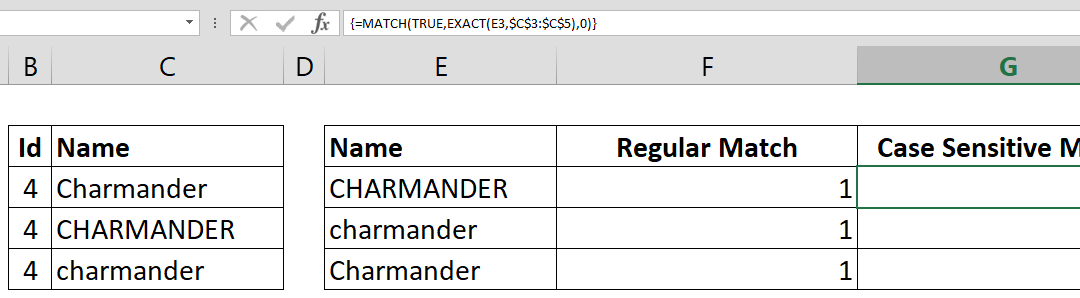## How to lookup across two tables with VLOOKUP and IF functions

How to lookup across two tables by a condition is a combination of the VLOOKUP and the IF functions. The IF function handles which table and VLOOKUP handles what to search for. Syntax =VLOOKUP(lookup value, IF(condition, first table if condition is TRUE, second table...## How to calculate monthly averages

This article shows you how to calculate monthly averages from a data table containing dates and values using AVERAGEIFS and EOMONTH functions. If you only want to calculate the number of days in a month, please refer to the article: How to find number of days in month...## How to find number of days in a month

This article shows you how to find number of days in a month to make your month-driven calculations dynamic. DAY and EOMONTH functions help us for this tip. Syntax =DAY(EOMONTH(Date, 0)) Steps Start with =DAY( Continue with EOMONTH( Select or type the range that...## How to make case sensitive match

If your data is case sensitive, you may have issues finding correct matches with lookup functions. This How to make case sensitive match article shows you how to combine MATCH and EXACT functions to achieve case sensitive matching. Syntax { =MATCH(TRUE, EXACT(search...## How to calculate Basic Tax Rate with VLOOKUP

The tax brackets can be confusing to work with. Different ‘levels’ of your income needs to be multiplied with a different percentage to find the total. This can be done with the conventional Excel formulas like IF and a bunch of others, but that’s the long and hard to...## How to check if a value exists in a list

The logical tests and conditional checks have important role in Excel models. Potential errors can be detected and handled by using the right logical tests. If you know How to check if a value exists in a list, you can use this logical statement to detect and...